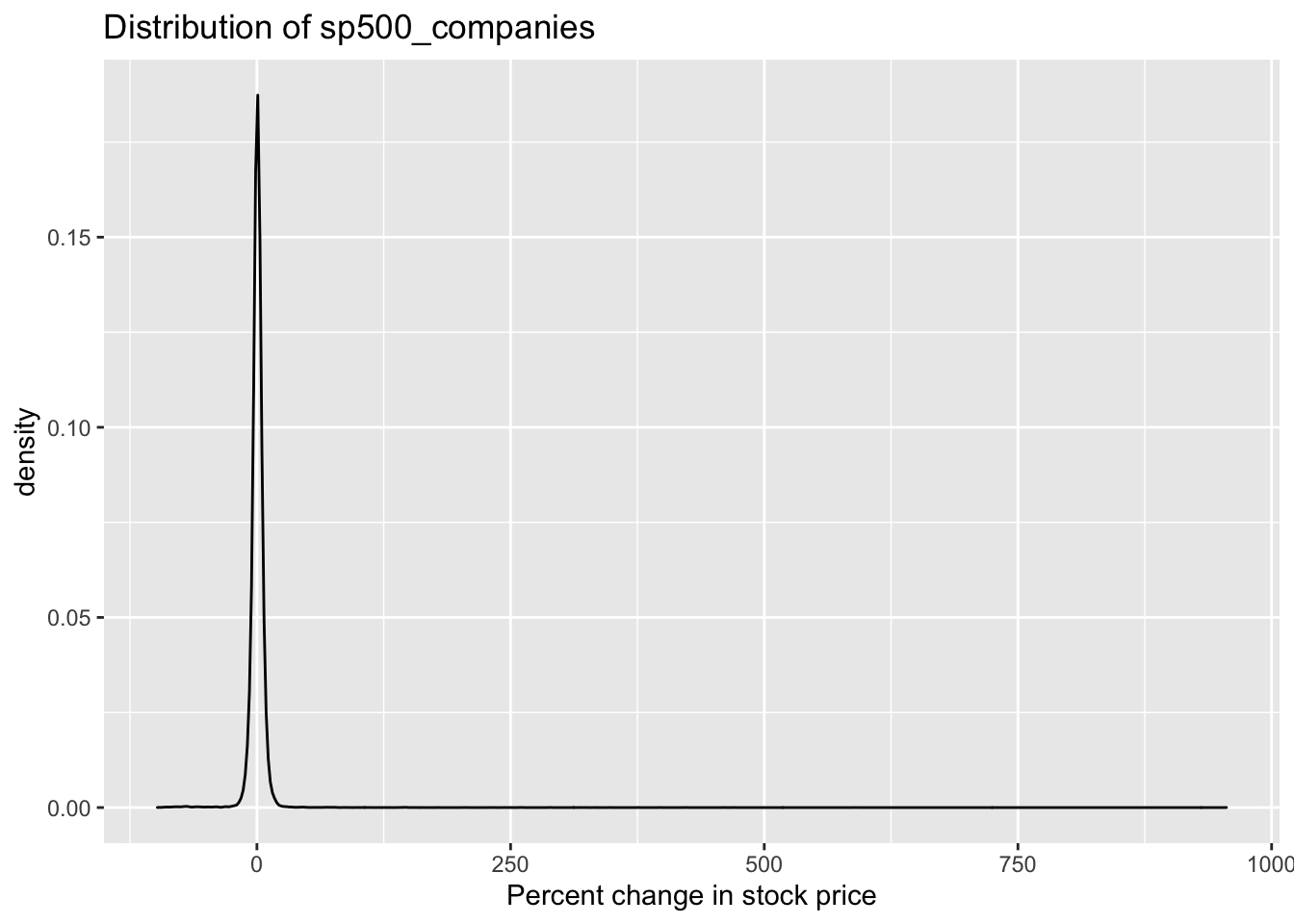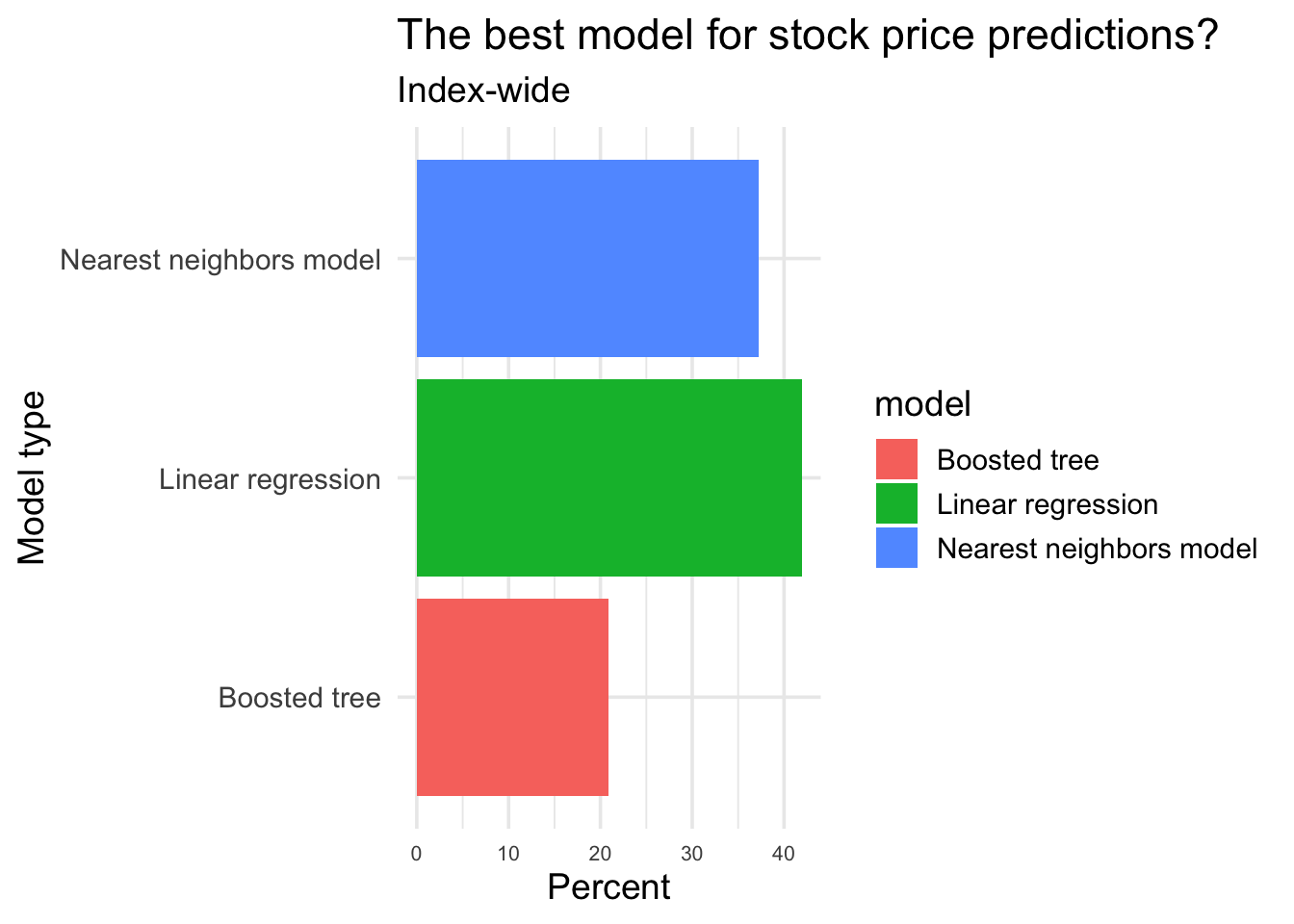## Overview

One of the most tantalizing challenges in modeling is trying to model stock prices - the more accurately you can predict a normally unpredictable marketplace, the more great trades you can make that could lead to financial success. Banks, brokerages, and other players in the stock market have spent years (especially now in the age of robo-investing) trying to accurately predict the markets.

In this project, I’ll do this on a smaller and more narrow scale. I’ll focus on the stocks that were in the S&P 500 from 2013 to 2017, and instead of trying to predict a stock’s individual price, I’ll focus on getting a sense of what type of model works best for the index as a whole and for the sectors that make it up.

To do this, I’ll create three models for each company in the index, focus them all on predicting the percent change in a stock’s price at two-week intervals, and then compare the models to find the most accurate one for each company. Then, I’ll aggregate this data to get a sense of which model works best on an index-wide level, and on an individual sector-level.

By doing this, we can answer one key question in creating a highly accurate stock model - which model should you use?

## Key questions

• What model (linear regression, boosted tree, nearest neighbors) provides the most accurate predictions for the percent change in stock price across the S&P 500?

• What model (linear regression, boosted tree, nearest neighbors) provides the most accurate predictions for the percent change, in each sector?

## Setting up the data

To setup the data, I imported the stock data for the 500 individual companies (all_stocks_5yr.csv, which becomes sp500_companies) and the data that has company details like the name and sector (constituents.csv, which became company_names).

After importing the data, I turned the dates int o R-understandable values, then separated the company stock data into training data (the data from 2013 through 2016) and the final testing data (2017).

After combining the individual company stock data with the company details, I moved on to setting the models up.

Here’s some information on the sp500_companies data set:

``````#checking to see that everything looks OK

sp500_companies %>%
skimr::skim_without_charts()``````
 Name Piped data Number of rows 411219 Number of columns 9 _______________________ Column type frequency: character 3 Date 1 numeric 5 ________________________ Group variables None

Variable type: character

skim_variable n_missing complete_rate min max empty n_unique whitespace
Name 0 1 1 5 0 427 0
Name.y 0 1 5 38 0 427 0
Sector 0 1 6 22 0 11 0

Variable type: Date

skim_variable n_missing complete_rate min max median n_unique
date 0 1 2013-02-08 2016-12-30 2015-01-29 982

Variable type: numeric

skim_variable n_missing complete_rate mean sd p0 p25 p50 p75 p100
open 10 1 77.11 73.94 1.62 39.35 59.95 89.62 845.79
high 7 1 77.82 74.60 1.69 39.76 60.52 90.42 847.21
low 7 1 76.38 73.22 1.61 38.98 59.36 88.85 840.60
close 0 1 77.13 73.93 1.62 39.37 59.96 89.67 844.36
volume 0 1 4650837.31 9465922.65 0.00 1080972.00 2133655.00 4577012.50 618237630.00
``````#basically no missing values

sp500_companies %>%
mutate(date = ymd(date)) %>%
filter(mod(week(date), 2) == 0) %>%
filter(wday(date) == 2) %>%
mutate(price_change = (close/lag(close) - 1) * 100) %>%
ggplot() +
geom_density(aes(price_change)) +
labs(title = "Distribution of sp500_companies", x = "Percent change in stock price")``````
``## Warning: Removed 1 rows containing non-finite values (stat_density).``Out of the 411,219 observations in sp500_companies, there are a total of 10 rows with missing data.

Looking at the density chart (having made the same data modifications that I used for analysis), the distribution seems to be normal, with all of the percent changes in price in a small window of values.

Now that we’ve looked at the data, we can set up the models we’ll use to analyze it.

## Setting up the models

The models I’m using for this EDA are: - A simple linear regression model, using the “lm” engine, - a boosted tree algorithmic model, using the “xgboost” engine, - and a k-nearest neighbors model, using the “kknn” engine.

The trees, mtry and learn_rate parameters for the boosted tree model were set to be tuned, along with the neighbors parameter for the nearest neighbor model. Grids were then made for these two models to find the best values for these parameters.

Now, let’s analyze all of the companies.

## Setting up the function and for loop

To apply these three models to each of the 500 companies, we have to set up: - A function, that takes a company and a model as an input, and then runs the best version of that model (based on the model’s RMSE value) on the company’s stock data. This function then returns the mean absolute error, or the average difference between the predicted percent change and the company’s actual percent change in 2017. - A for loop, which will put every company & model combination through the above function, and will output that combination’s mean absolute error.

This was already done (along with all of the data processing and model setup above) in the “data_model_prep_.R” R script (it took eight and a half hours!). Now all we have to do is graph our results.

## Cleaning up and presenting our results

To graph our results, we’ll import the already processed company details dataset, company_names.csv, along with the finished function and for loop output, compare_models.rds.

Then, we’ll filter the data to only get the most accurate company-model combinations (by looking at the combos with the lowest mean absolute error). Once we have that, we can graph the results on a sector-by-sector basis, and the results looking at the stock index overall.

Without further ado, here are the graphs!

``````#importing the data from the previous R chunk

#selecting only the winning models (which model had the smallest average percent difference between real and predicted values)

#used rmse for model comparison
#mean absolute error to assess model

final_results <- compare_models %>%
group_by(X.Company.name.) %>%
filter(X0 == min(X0))

#renaming the variables so they have less gross names
final_results <- final_results %>% rename("avg_dif" = X0, "ticker" = X.Company.name., "model" = X.Model.)

#joining results with dataset that contains sector information
final_results <- inner_join(final_results, company_names,
c("ticker" = "Symbol"))

#creating percent information based on sectors
final_results_sector <- final_results %>%
group_by(Sector, model) %>%
summarise(count = n()) %>%
mutate(perc = count/sum(count))

#creating percent information based on the entire dataset
final_results_ind <- final_results %>%
group_by(model) %>%
summarise(count = n()) %>%
mutate(perc = count/sum(count))

#graphing our results

#overall
ggplot(final_results_ind, aes(factor(model),y = perc*100, fill = factor(model))) +
geom_bar(stat = "Identity") +
labs(x = "Model type", y = "Percent", fill = "model", title = "The best model for stock price predictions?", subtitle = "Index-wide") +
theme_minimal(base_size = 14) +
theme(axis.text.x = element_text(size = 8)) +
coord_flip()````````````#by sector
ggplot(final_results_sector, aes(factor(Sector),y = perc*100, fill = factor(model))) +
geom_bar(stat = "Identity") +
labs(x = "Sector", y = "Percent", fill = "model", title = "The best model for stock price predictions?", subtitle = "Sector by sector") +
theme_minimal(base_size = 14) +
theme(axis.text.x = element_text(size = 8)) +
coord_flip()``````